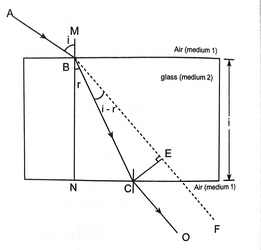Lateral Shift and Types of DepthsLateral shift (displacement) ﻿

A ray of light incidents at one face of slab of thickness 't' and it emerges into same medium after refraction through the slab, the emergent ray becomes parallel to incident ray. So deviation of emergent ray is zero. But the emergent ray gets displaced laterally by a distance 'd'. The perpendicular distance between incident ray and emergent ray called lateral shift.

The lateral displacement is discussed for the incident ray by considering the passage of light ray from air (medium 1) to glass (medium 2) to air (medium 1). As shown in figure, suppose a ray of light AB travelling in air is refracted along BC into glass and emerges into air along CD.

Let 'i' and 'r' are the angle of incidence in air and angle of refraction in glass. The perpendicular distance CE between the emergent ray CD and the produced incident ray AB gives the measure of lateral displacement for incident ray produced by glass.

Now, in right angled triangle BEC,Thus, lateral displacement depends on the thickness of the medium, material of the medium and the angle of incidence. The second figure above shows the variation of lateral shift with the angle of incidence.

When i=r, which is possible for normal incidence (i=0°), d=0. There will be no lateral shift of the emergent ray if the light is incident normally on the glass (medium 2).

When, i=90°, i.e. for grazing incidence,

d= t secr

And,  d= sin(90°-r)

or,  d= t secr. cosr = t.

When light is incident parallel to the glass slab, the lateral displacement (shift) is equal to the thickness of the glass (medium 2).

Real and apparent depth.

Due to refraction, the depth of an object lying inside an optically denser medium appears to be less than its real depth.

Let, an object O at a distance t below the surface PQ of a transparent medium such as glass, which has absolute refractive index ng

A ray OA from O perpendicular to surface PQ passes straight into the air. A ray OB very close to OA is refracted into air along BD. If BD is produced to meet OA at I, which is the position of virtual image of the object O i.e., an observer viewing O directly overhead sees it in the postion of I. Hence, A1 is the apparent depth of the object where as its real depth is AO. Let i and r are respectively the angles made by the rays OB in glass and BD in air with the normal at B. Then, for refraction at B, from glads to air, we have,

ng sini = na sinr

Where na is the absolute refractive index of air which is unity.

Here, <BOA = i (Alternate angle)and <BIA = r (Corresponding angles)

From right angled triangle AOB,Since, the observer is directly above O, the angles i and r are small and the rays OB and IB are very close to OA i.e. B is very close to A, then.The distance OI is called the displacement d of the object. If t be the real depth of the object.

Then OA = tTotal internal reflection

Total internal reflection may be defined as the phenomenon of reflection of light that takes place, when a ray of light travelling in a denser medium gets incident at the interface of two media at an angle greater than the critical angle for that pair of media.The critical angle (C) for a pair of media maybe defined as the angle of incidence in the denser medium, for which the angle of refraction in the rarer medium is 90°.

In the figure above, when the critical angle of the medium is smaller than the angle of incidence the light reflects back to the same medium. Whereas in other condition like when angle of incidence is smaller than the critical angle there is refraction light and when there is angle of incidence just equal to the critical angle the ray of light just passes through the border of the two media.

Condition for total internal reflection

1. Light ray must travel from denser medium to rarer medium.

2. The angle of incidence in denser medium must be greater than the critical angle for these two media.

A ray of light OA is passing from one medium of refractive index n1 to another medium of refractive index n2 , where n1>n2. Let C be the angle of incidence in the first medium for which the angle of refraction in the second medium is 90°. The angle of incidence is the critical angle for medium 1 and medium 2 boundary.

Now applying Snell's law, in the form of  nsin θ1=n2 sin θnsin θ=nsin 90°

n1 sinC = n2

SinC = n2 / n1

If the second medium is air,Absolute refractive index of glass is 1.5. Hence, critical angle if glass-air similarly, interface is 41.8°.

Similarly, the critical angle for glads water interface is 62.7°.